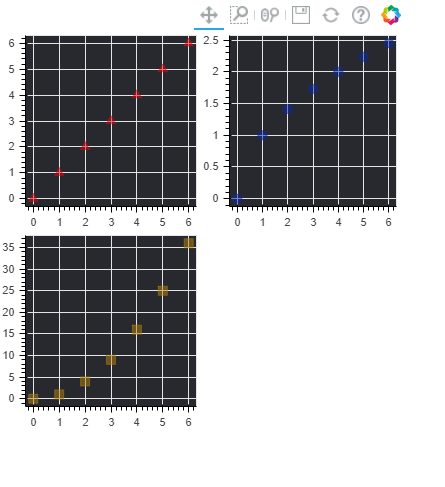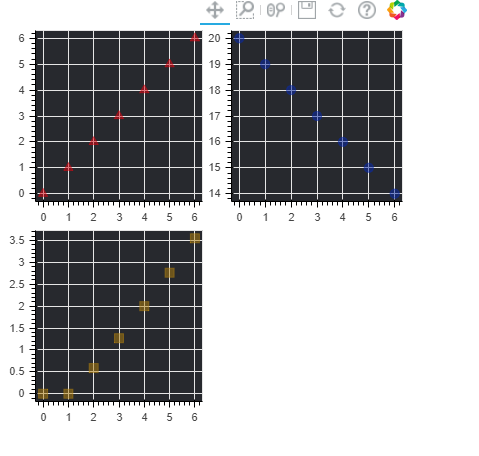Related Articles

# Bokeh – grid layout of plots

• Last Updated : 25 Feb, 2021

Bokeh includes several layout options for arranging plots and widgets. They make it possible to arrange multiple components to create interactive dashboards or data applications. The layout functions let you build a grid of plots and widgets. You can nest as many rows, columns, or grids of plots together as you’d like. In addition, Bokeh layouts support a number of “sizing modes”. These sizing modes allow plots and widgets to resize based on the browser window.

Grid Layout :

Grid layout basically means all your plots will be in a grid-based format and those who have used some basic css, bootstrap can easily understand what grid represents.

How to install bokeh :

`pip install bokeh`

Basic Approach Of All Examples :

• First calculate List1, List2, List3 these three are list obtained by performing some operations on currentList and all three of them will have the same length and List1 is the same as of currentList (List1=currentList).
• List2 and List3 will be different operations performed on currentList and in each example, the operations will be different.
• In the end after calculating List2, List3 we will plot three of them List1, List2, List3 using Bokeh-grid Layout.
• Grid Layout basically means we will represent (currentList, List1),(currentList, List1), (currentList, List1) in form of a grid, and those who know bootstrap and css can relate to the meaning of grid here very easily.

Example 1:

• Here we will give currentList values from 0 to 6 thats why we use range(7) then we passed this in the list() constructor and then we assigned it to List1.
• Now List2 will denote the square root of every element in currentList List2=[i**0.5 for i in currentList] and List3 will denote the square of every element of currentList List3=[i**2 for i in currentList] and we know in python we can easily perform power operation using ** operator as we have used **0.5 for calculating square root and **2 for calculating square.
• For (currentList, List1) plot we will use the circle to represent the point on the plot and for (currentList, List2) triangle and (currentList, List3) square. And the output will be generated in GFG.html as mentioned in the code.
• f1 = figure(background_fill_color=”#27292e”) in background_fill_color represents the background color you can customize it also.
• f1.triangle(currentList, y0, size=9, alpha=0.5, color=”#de040f”) here size =9 means the size of the point on the plot and color means color of point and alpha means how much darker or light you want , you can also try to change these attributes to understand better.
• Now show all plots in grid layout show( gridplot([[f1, f2], [f3, None]], plot_width=200, plot_height=200)).

Syntax :

```output_file("GFG.html")
currentList = list(range(7))

# writing logics for List1,List2,List2
# creating plots with these atrributes
# color,backgroundcolor,aplha ,size
# splots are f1,f2,f3
# using grid layout
show(gridplot([[f1, f2], [f3, None]], plot_width=200, plot_height=200))```

Below is the implementation:

## Python3

 `# python program for bokeh grid layout``from` `bokeh.io ``import` `output_file, show``from` `bokeh.layouts ``import` `gridplot``from` `bokeh.plotting ``import` `figure`` ` `# output will be produced in GFG.html``output_file(``"GFG.html"``)`` ` `# list will contain from 0 to 6``currentList ``=` `list``(``range``(``7``))`` ` `# y0 is equals to x no change``List1 ``=` `currentList`` ` `# y1 square root of x``List2 ``=` `[i``*``*``0.5` `for` `i ``in` `currentList]`` ` `# y2 square  of x``List3 ``=` `[i``*``*``2` `for` `i ``in` `currentList]`` ` `# now creating plots f1,f2,f3``f1 ``=` `figure(background_fill_color``=``"#27292e"``)``f1.triangle(currentList, List1, size``=``9``, alpha``=``0.5``, color``=``"#de040f"``)`` ` `f2 ``=` `figure(background_fill_color``=``"#27292e"``)``f2.circle(currentList, List2, size``=``9``, alpha``=``0.5``, color``=``"#0828a8"``)`` ` `f3 ``=` `figure(background_fill_color``=``"#27292e"``)``f3.square(currentList, List3, size``=``9``, alpha``=``0.5``, color``=``"#b3810c"``)`` ` `# using grid layout on f1,f2,f3 plots``show(gridplot([[f1, f2], [f3, ``None``]], plot_width``=``200``, plot_height``=``200``))`

Output :Example 2 :

• Note that in this example we changed only the logic to calculate List2,List3 and rest other things are same.
• Here we will give currentList values from 0 to 6 that’s why we use range(7) then we passed this in list() constructor and then we assigned it to List1.
• Now lets calculate List2,List3 here List2 will represent the absolute difference between 20 and every element of currentList List2=[abs(20-i) for i in currentList] and List3 represents absolute difference between every element of currentList and square root of that element List3=[abs(i-i**0.5) for i in currentList].
• Why we used abs() function because in some cases it might be possible to get a negative value to convert it into positive we use abs().
• Now again we will use grid on three plots (currentList,List1), (currentList,List2) and (currentList,List3).
• Here we used the circle to represent a point on the first plot and a triangle on the second for the third we used squares.
• The output will be shown on GFG.html as written in the code.
• f1 = figure(background_fill_color=”#27292e”) in background_fill_color represents the background color you can customize it also.
• f1.triangle(currentList, y0, size=9, alpha=0.5, color=”#de040f”) here size =9 means the size of the point on the plot and color means color of point and alpha means how much darker or light you want , you can also try to change the these attributes to understand better.
• Now show all plots in grid layout show( gridplot([[f1, f2], [f3, None]], plot_width=200, plot_height=200)).

Syntax :

```output_file("GFG.html")
currentList = list(range(7))

# writing logics for List1,List2,List3
# creating plots with these atrributes
# color,backgroundcolor,aplha,size
# plots are f1,f2,f3
# using grid layout
show( gridplot([[f1, f2], [f3, None]], plot_width=200, plot_height=200))```

Below is the implementation:

## Python3

 `# python program for bokeh grid layout``from` `bokeh.io ``import` `output_file, show``from` `bokeh.layouts ``import` `gridplot``from` `bokeh.plotting ``import` `figure`` ` `# output will be produced in GFG.html``output_file(``"GFG.html"``)`` ` `# list will contain from 0 to 6``currentList ``=` `list``(``range``(``7``))`` ` `# List1 is equals to currentList no change``List1 ``=` `currentList`` ` `# List2 square root of currentList``List2 ``=` `[``abs``(``20``-``i) ``for` `i ``in` `currentList]`` ` `# List3 square  of currentList``List3 ``=` `[``abs``(i``-``i``*``*``0.5``) ``for` `i ``in` `currentList]`` ` `# now creating plots f1,f2,f3``f1 ``=` `figure(background_fill_color``=``"#27292e"``)``f1.triangle(currentList, List1, size``=``9``, alpha``=``0.5``, color``=``"#de040f"``)`` ` `f2 ``=` `figure(background_fill_color``=``"#27292e"``)``f2.circle(currentList, List2, size``=``9``, alpha``=``0.5``, color``=``"#0828a8"``)`` ` `f3 ``=` `figure(background_fill_color``=``"#27292e"``)``f3.square(currentList, List3, size``=``9``, alpha``=``0.5``, color``=``"#b3810c"``)`` ` `# using grid layout on f1,f2,f3 plots`` ` `show(gridplot([[f1, f2], [f3, ``None``]], plot_width``=``200``, plot_height``=``200``))`

Output :Attention geek! Strengthen your foundations with the Python Programming Foundation Course and learn the basics.

To begin with, your interview preparations Enhance your Data Structures concepts with the Python DS Course. And to begin with your Machine Learning Journey, join the Machine Learning – Basic Level Course

My Personal Notes arrow_drop_up